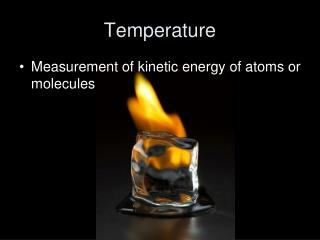DownloadDownload PresentationTemperature

# Temperature

Télécharger la présentation## Temperature

- - - - - - - - - - - - - - - - - - - - - - - - - - - E N D - - - - - - - - - - - - - - - - - - - - - - - - - - -
##### Presentation Transcript

1. Temperature • Measurement of kinetic energy of atoms or molecules

2. Fahrenheit (°F) Celsius (°C) Based on boiling & freezing point of water Temperature Scales Water Boils Water Freezes Absolute Zero 373 212 100 32 273 0 - 452 - 273 0 Kelvin (K)

3. Kelvin temperature scale • Based on absolute zero • Absolute zero is when all motion of atoms stop! 273 + °C = K

4. Accuracy • How close a measured value is to the accepted value or target • Precision • How close a series of measurements are to one another

5. Accurate = means close to the bull’s eye Precise = means shots are close to one another

6. Practice • Density of water is 1 g/mL • Student one • Accurate? • Precise? • Student two • Accurate? • Precise?

7. Percent Error • Ratio of error to the accepted value • Can be positive or negative • We like to within 5% 

8. Percent Error Practice Problems 1. The boiling point of water is 100°C. During an experiment, water came to a boil at 97°C according to the thermometer that was being used. What is the percent error?

9. Percent Error Practice Problems 2. An experiment was performed to determine the density of water. The results of the experiment showed that water had a density of 1.15 g/mL(real value is 1.0 g/mL). What is the percent error?

10. Percent Error Practice Problems 3. An experiment was conducted to find the mass of one mole of carbon atoms. The results of the experiment showed that a mole of carbon atoms has a mass of 15.78g. The actual value is 16 grams. What is the percent error?

11. Percent Error Practice Problems 4. An experiment performed to determine the density of lead yields a value of 10.95 g/cm3. The actual value for the density of lead is 11.342 g/cm3. Find the percent error.

12. Percent Error Practice Problems 5. Find the percent error in a measurement of the boiling point of bromine if the experimental value is 40.6°C and the actual value is 59.35°C.